# Tree Recursion

## Tree Recursion in C Language with Examples

In this article, I am going to discuss Tree Recursion in C Language with Examples. Please read our previous article, where we discussed Head Recursion in C Language with Examples. First, we will compare Linear Recursion with Tree Recursion. Recursion can be broadly divided into 2 types

1. Linear Recursion.
2. Tree Recursion.
##### Linear Recursion in C Language:

A function that calls itself, is a recursive function. If it is calling itself only one time, and before and after the function call, if we are doing something, then it is a linear recursion. Please have a look at the below example which is an example of a linear recursive function. Here, before and after the function call i.e. fun(n-1), we have statements, so it is a linear recursive function.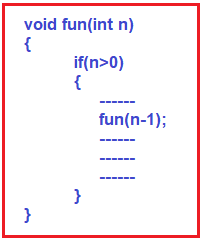##### Tree Recursion in C Language:

A function that calls itself, is a recursive function. If it is calling itself more than one time, then it is a tree recursion. Please have a look at the below example which is an example of a tree recursive function. As you can see fun is calling itself 2 times (more than 1 time). In our example, we have shown the recursive call like 2 times, but it can also be more than 2 times.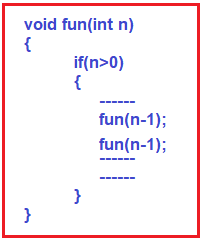In tree recursion fun(n-1) (1st recursive call) will perform all its task at the time of calling and fun (n-1) (2nd recursive call) will perform all its task at the time of returning.

##### Example: Tree Recursion in C Language

As you can see in the below example, within the if block the first statement is the print statement, when we call the function then it first prints the statement. After that there are two recursive calls, the second recursive call will execute when the first call is finished.

```int main() {
int x = 3;
TreeRecursion(x);
}
void TreeRecursion(int n) {
if (n > 0) {
printf("%d\n", n);
TreeRecursion(n - 1);
TreeRecursion(n - 1);
}
}
```

As you can see in the above code, we have mainly three tasks that need to be performed within the fun function.

1. Print the value of n
2. fun function call 1st time with the reduced value of n.
3. fun function call 2nd time with the reduced value of n.

Let’s jump on the tracing part where we understand each and every step.

##### Tracing Tree and Memory Allocation of Tree Recursion in C Language:

Let’s trace our recursion tree step by step

##### Step1: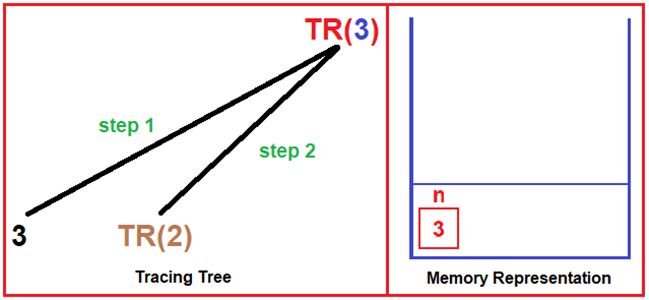Output: 3

Tracing Tree: As you can see in the above diagram, here we call our recursive function TreeRecursion(n) with a parameter of 3 i.e., TR (3). Now, it checks if 3 > 0? yes, then it will execute the next line which is our print statement which will print 3. The next two statements are the recursive calls. The first one will execute TR (3 – 1). Both calls cannot execute at the same time, for the execution of the second statement, the first call should be finished then only control goes to the second call.

Memory Representation: For the TR (3) call, one activation record is created and the value of n will be 3.

##### Step2: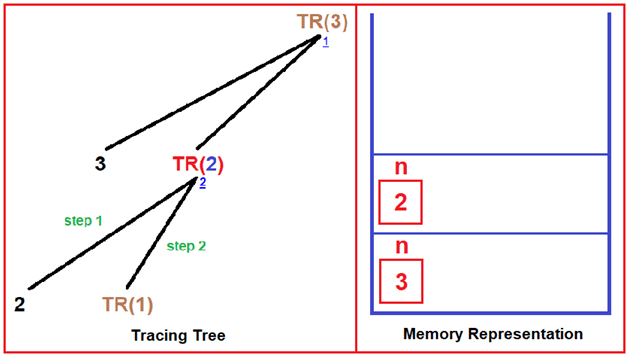Output: 3 2

Tracing Tree: Now TR (2) call check for the condition if 2 > 0? yes, then it executes the print statement which prints 2. The next step is again recursive call, the next one executes when the first is finished. So here it calls TR (2 – 1). Here underlined number shows the function calling sequence.

Memory Representation: For the TR (2) call, again the activation record is created and the value of n will be 2.

##### Step3: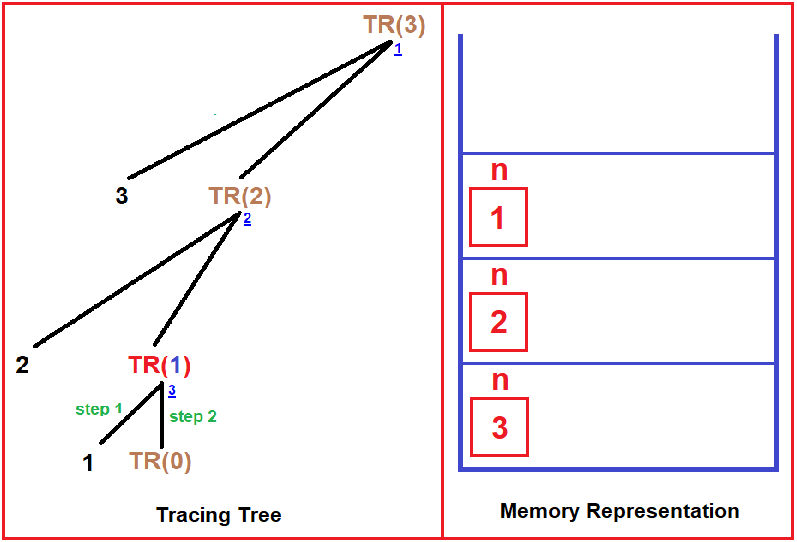Output: 3 2 1

Tracing Tree: Now same steps perform in TR (1) call. First it checks condition if 1 > 0? Yes, then it prints 1. The next steps are again two recursive calls. The first call will execute TR (1 – 1) and when it terminates then only the second will execute.

Memory Representation: For the TR (1) call, the activation record is created and the value of n will be 1.

##### Step4: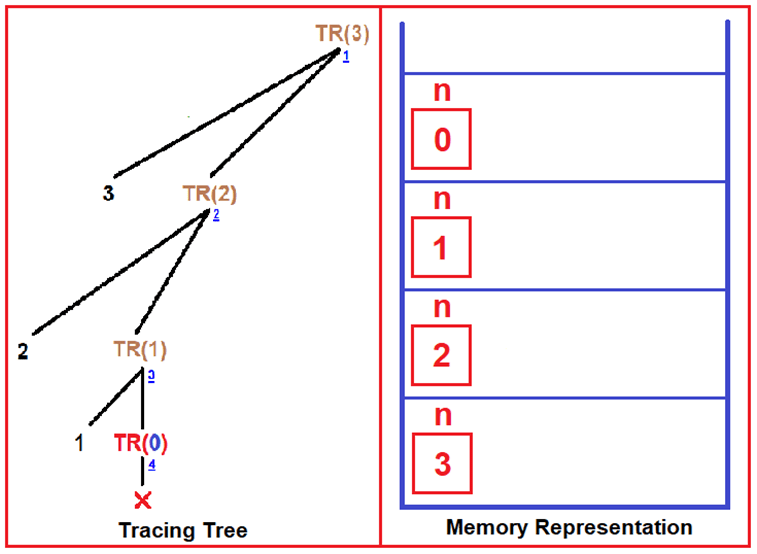Output: 3 2 1

Tracing Tree: In TR (0) call, it doesn’t satisfy our condition which is if 0 > 0, No, then here function terminates and the control goes back to the previous call which is TR (1).

Memory Representation: For the TR (0) call, the activation record is created and the value of n will be 0.

##### Step5: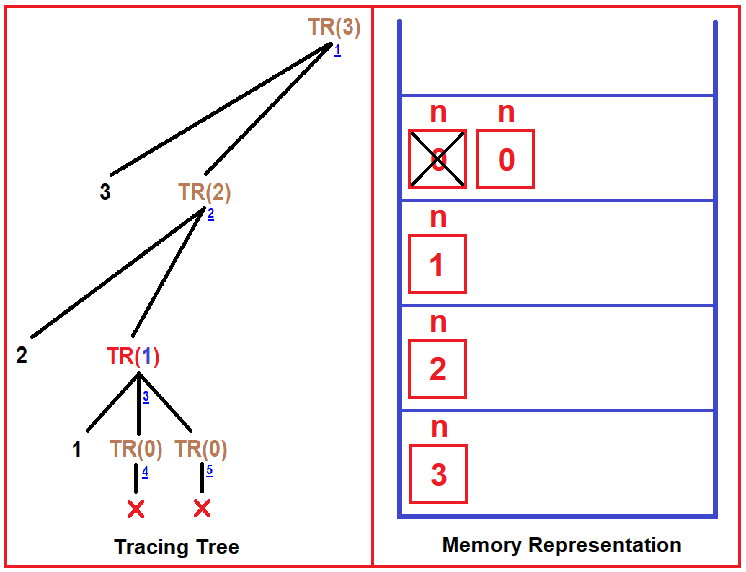Output: 3 2 1

Tracing Tree: Now back to TR (1) call and here it will execute its second recursive call which is TR (1 – 1) which is TR (0). As we discussed TR (0) in the previous step it will terminate because it doesn’t satisfy the condition. Here TR (1) call is completed and now control goes back to TR (2) call.

Memory Representation: As the function terminates, so, n(0) activation record is deleted and then a fresh TR (0) is made so again new activation record of n (0) will be created in the stack.

##### Step6: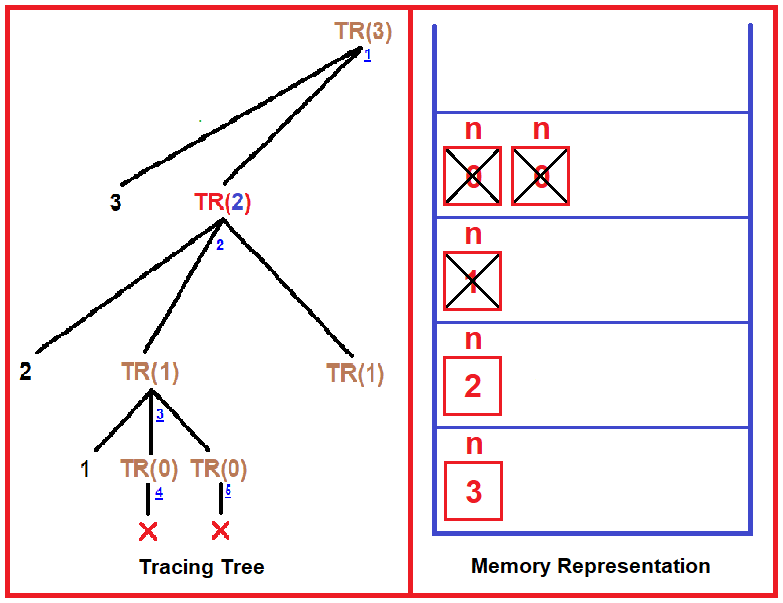Output: 3 2 1

Tracing Tree: Now TR (2) executes its second recursive call which is TR (2 – 1) which is TR (1).

Memory Representation: Now, here both TR (0) calls have finished so the activation record is also deleted, now control goes back to TR (1) call which all three steps (print, two recursive calls) have completed, so activation record n (1) will also be deleted and control goes back to TR (2) and then it executes it second recursive call.

##### Step7: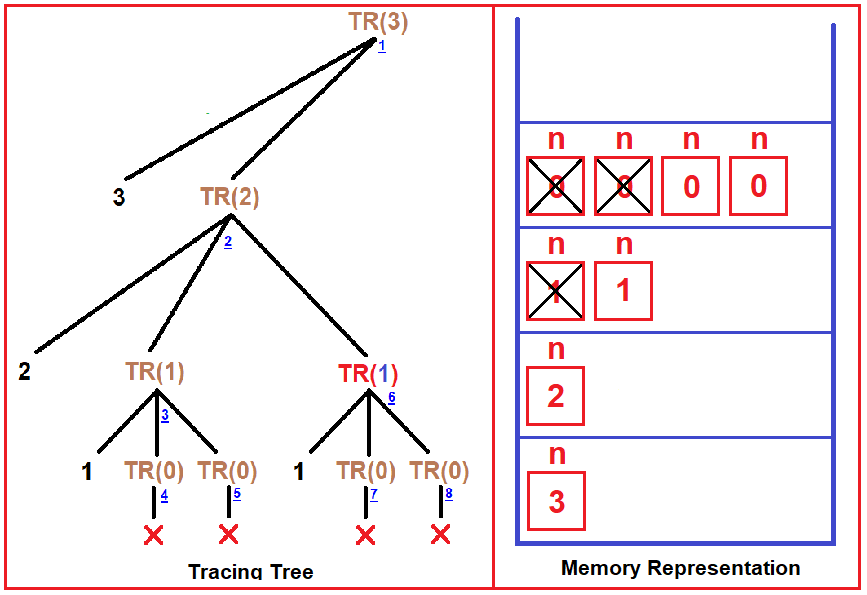Output: 3 2 1 1

Tracing Tree: Now again control shift to TR (1) which prints 1 and executes its two recursive calls which are TR (0) and we know it terminates here. Now TR (0) is terminated, control back to TR (1) whose all calls have finished and control goes back to TR (2), and all calls of TR (2) are also finished. Now it goes back to TR (3).

Memory Representation: Now, TR (2) execute its last recursive call TR (1) which again creates another activation record n (1), then further TR (1) creates other two activation records of n (0).

##### Step8: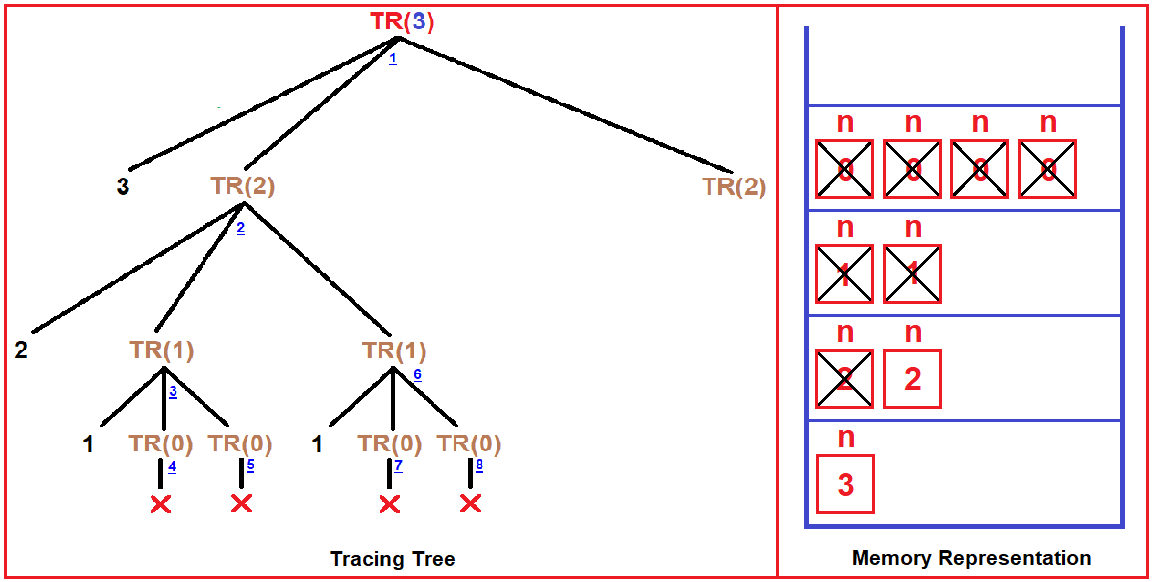Output: 3 2 1 1

Tracing Tree: In TR (3) call, it executes its second recursive call which is TR (3 – 1). As we discussed in previous steps about TR (2) calls, it will execute in the same manner.

Memory Representation: As both TR (0) calls have finished so activation record will delete and the control goes to TR (1), here TR (1) all calls have finished. Now it returns to TR (2), again it’s all calls have finished and its activation record will delete, now control goes back to TR (3) which execute its second recursive call TR (2) which create another activation record of n (2) in the stack.

##### Step9: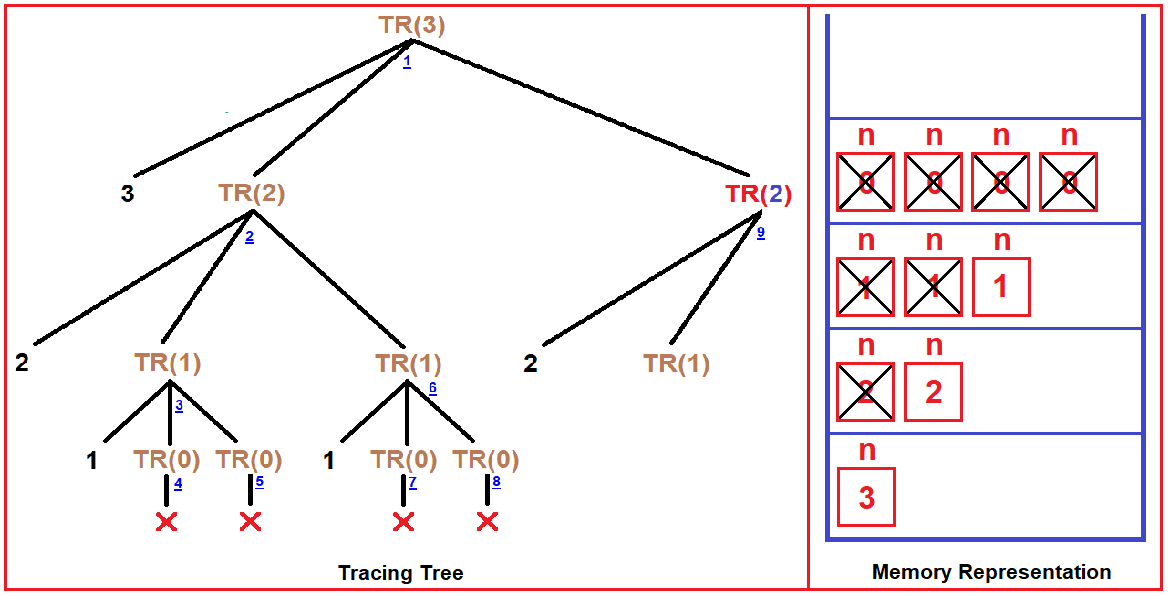Output: 3 2 1 1 2

Tracing Tree: The right-side TR (2) call executes its two recursive calls i.e., TR (1). The first call will execute.

Memory Representation: Here, TR (2) executes its TR (1) call.

##### Step10: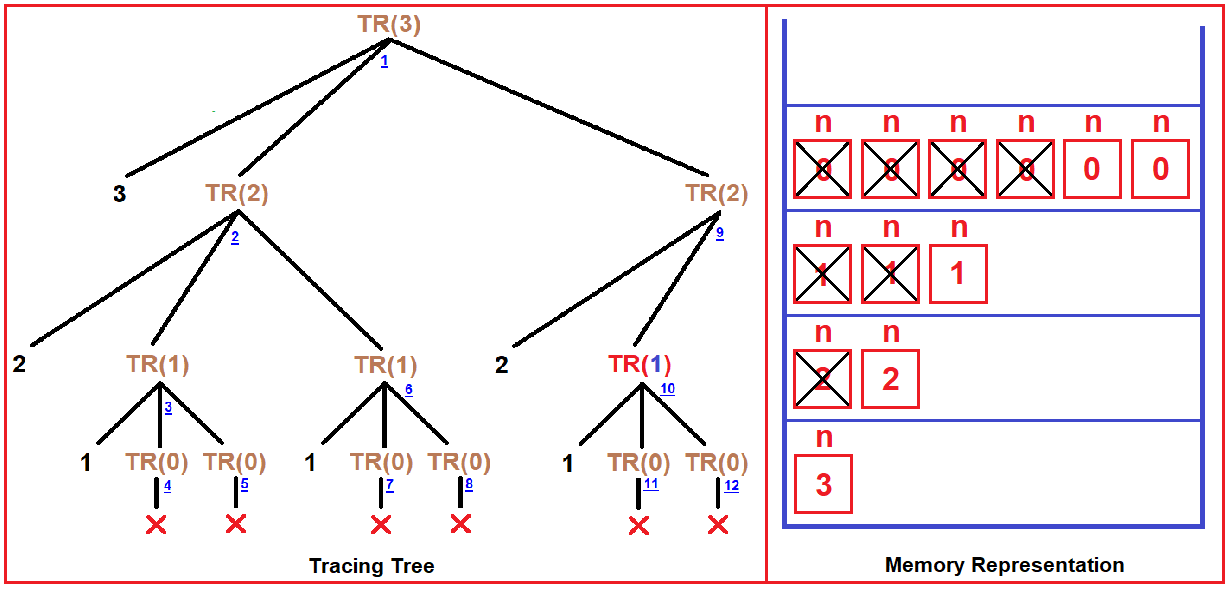Output: 3 2 1 1 2 1

Tracing Tree: As we discussed in previous steps that how TR (1) executes its next two recursive calls and at TR (0) they terminate and control goes back to the previous call.

Memory Representation: Here, two activation records will create of n (0).

##### Step11: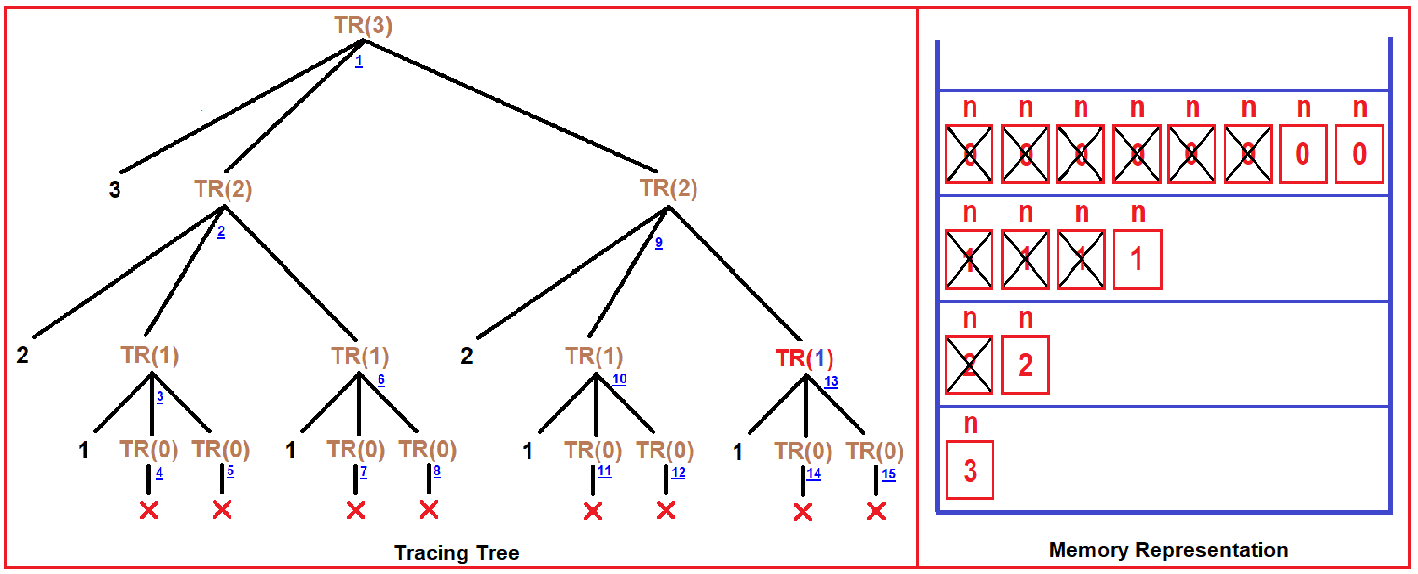Output: 3 2 1 1 2 1 1

Tracing Tree: This is our final tree diagram which executed all the calls of all recursive functions. Here TR(n) function ends.

Memory Representation: Here, another call of TR (1) created another two activation records of n (0).

##### Step12: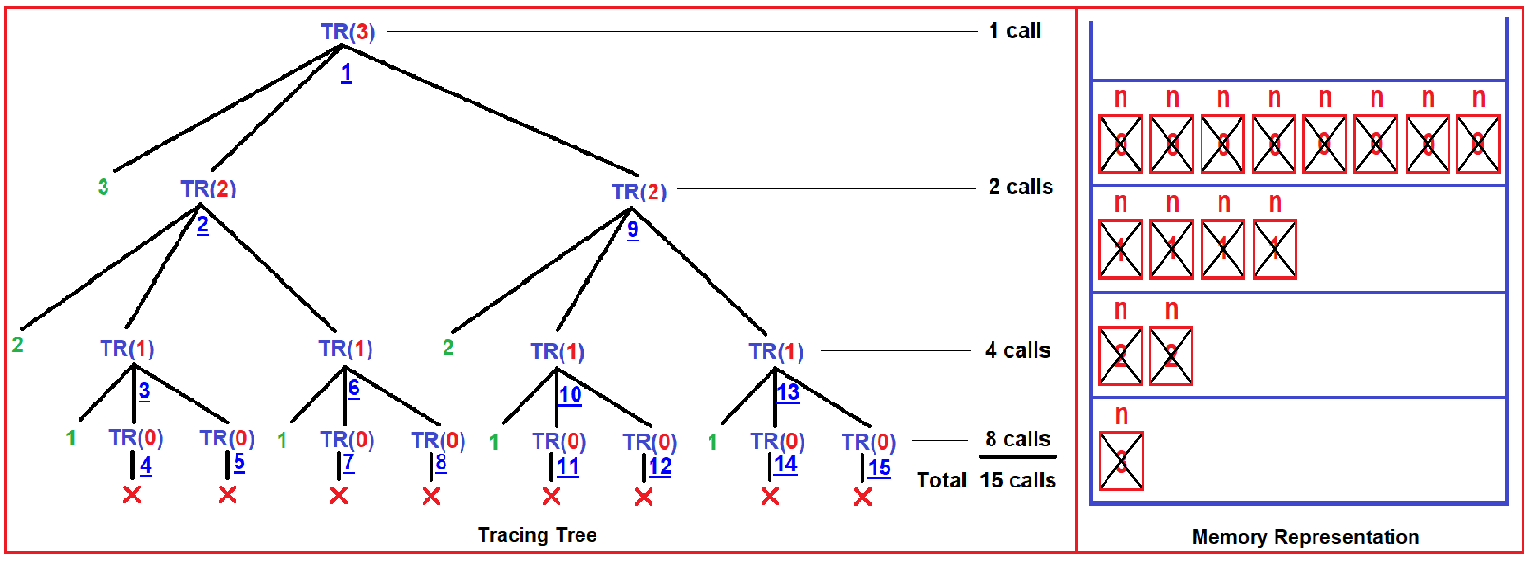Output: 3 2 1 1 2 1 1

Tracing Tree – In the above example, there are total 15 function calls.

So, 1 + 2 + 4 + 8 = 15 calls (G.P –Geometric Progression series)

We can write the above equation as,

20 + 21 + 22 + 23 = 23+1 – 1

20 + 21+ 22 + … + 2n+1 – 1

Time Complexity of this function is O(2n).

Memory Representation: above diagram shows the whole activation record. Here total number of activation record = total number of recursive calls.

##### Tree Recursion Example in C Language:
```#include <stdio.h>
void TreeRecursion(int n) {
if (n > 0) {
printf("%d\n", n);
TreeRecursion(n - 1);
TreeRecursion(n - 1);
}
}
int main() {
int x = 3;
TreeRecursion(x);
}
```

Output: 3 2 1 1 2 1 1

In the next article, I am going to discuss Indirect Recursion in C Language with Examples. Here, in this article, I try to explain Tree Recursion in C Language with Examples and I hope you enjoy this Tree Recursion in C Language with Examples article.